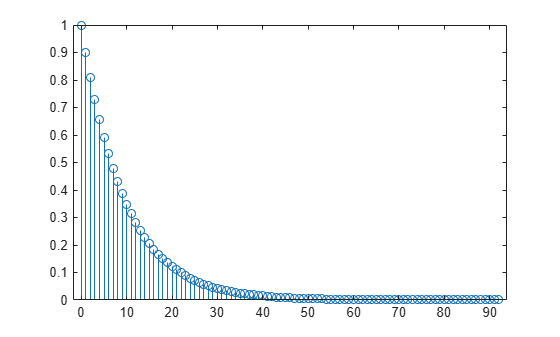impzlength

Impulse response length

Description

example

len = impzlength(b,a) returns the impulse response length for the causal discrete-time filter with the rational system function specified by the numerator, b, and denominator, a, polynomials in z–1. For stable IIR filters, len is the effective impulse response sequence length. Terms in the IIR filter’s impulse response after the len-th term are essentially zero.

example

len = impzlength(sos) returns the effective impulse response length for the IIR filter specified by the second order sections matrix, sos. sos is a K-by-6 matrix, where the number of sections, K, must be greater than or equal to 2. If the number of sections is less than 2, impzlength considers the input to be the numerator vector, b. Each row of sos corresponds to the coefficients of a second order (biquad) filter. The ith row of the sos matrix corresponds to [bi(1) bi(2) bi(3) ai(1) ai(2) ai(3)].

example

len = impzlength(d) returns the impulse response length for the digital filter, d. Use designfilt to generate d based on frequency-response specifications.

len = impzlength(___,tol) specifies a tolerance for estimating the effective length of an IIR filter’s impulse response. By default, tol is 5e-5. Increasing the value of tol estimates a shorter effective length for an IIR filter’s impulse response. Decreasing the value of tol produces a longer effective length for an IIR filter’s impulse response.

Examples

collapse all

Create a lowpass allpole IIR filter with a pole at 0.9. Calculate the effective impulse response length. Obtain the impulse response. Plot the result.

b = 1;
a = [1 -0.9];
len = impzlength(b,a)
len = 93
[h,t] = impz(b,a);
stem(t,h)h(len)
ans = 6.1704e-05

Design a 4th-order lowpass elliptic filter with a cutoff frequency of 0.4π rad/sample. Specify 1 dB of passband ripple and 60 dB of stopband attenuation. Design the filter in pole-zero-gain form and obtain the second-order section matrix using zp2sos. Determine the effective impulse response sequence length from the second-order section matrix.

[z,p,k] = ellip(4,1,60,.4);
[sos,g] = zp2sos(z,p,k);
len = impzlength(sos)
len = 80

Use designfilt to design a 4th-order lowpass elliptic filter with normalized passband frequency 0.4π rad/sample. Specify 1 dB of passband ripple and 60 dB of stopband attenuation. Determine the effective impulse response sequence length and visualize it.

d = designfilt('lowpassiir','FilterOrder',4,'PassbandFrequency',0.4, ...
'PassbandRipple',1,'StopbandAttenuation',60, ...
'DesignMethod','ellip');
len = impzlength(d)
len = 80
impz(d)Input Arguments

collapse all

Numerator coefficients, specified as a scalar (allpole filter) or a vector.

Example: b = fir1(20,0.25)

Data Types: single | double
Complex Number Support: Yes

Denominator coefficients, specified as a scalar (FIR filter) or vector.

Data Types: single | double
Complex Number Support: Yes

Matrix of second order sections, specified as a K-by-6 matrix. The system function of the K-th biquad filter has the rational Z-transform

${H}_{k}\left(z\right)=\frac{{B}_{k}\left(1\right)+{B}_{k}\left(2\right){z}^{-1}+{B}_{k}\left(3\right){z}^{-2}}{{A}_{k}\left(1\right)+{A}_{k}\left(2\right){z}^{-1}+{A}_{k}\left(3\right){z}^{-2}}.$

The coefficients in the Kth row of the matrix, sos, are ordered as follows.

$\left[\begin{array}{cccccc}{B}_{k}\left(1\right)\text{ }& {B}_{k}\left(2\right)\text{ }& {B}_{k}\left(3\right)& {A}_{k}\left(1\right)\text{ }& {A}_{k}\left(2\right)& {A}_{k}\left(3\right)\end{array}\right]$

The frequency response of the filter is the system function evaluated on the unit circle with

$z={e}^{j2\pi f}.$

Digital filter, specified as a digitalFilter object. Use designfilt to generate a digital filter based on frequency-response specifications.

Example: d = designfilt('lowpassiir','FilterOrder',3,'HalfPowerFrequency',0.5) specifies a third-order Butterworth filter with normalized 3-dB frequency 0.5π rad/sample.

Tolerance for IIR filter effective impulse response length, specified as a positive number. The tolerance determines the term in the absolutely summable sequence after which subsequent terms are considered to be 0. The default tolerance is 5e-5. Increasing the tolerance returns a shorter effective impulse response sequence length. Decreasing the tolerance returns a longer effective impulse response sequence length.

Output Arguments

collapse all

Length of the impulse response, specified as a positive integer. For stable IIR filters with absolutely summable impulse responses, impzlength returns an effective length for the impulse response beyond which the coefficients are essentially zero. You can control this cutoff point by specifying the optional tol input argument.

Algorithms

To compute the impulse response for an FIR filter, impzlength uses the length of b. For IIR filters, the function first finds the poles of the transfer function using roots.

If the filter is unstable, the length extends to the point at which the term from the largest pole reaches 106 times its original value.

If the filter is stable, the length extends to the point at which the term from the largest-amplitude pole is tol times its original amplitude.

If the filter is oscillatory, with poles on the unit circle only, then impzlength computes five periods of the slowest oscillation.

If the filter has both oscillatory and damped terms, the length extends to the greater of these values:

• Five periods of the slowest oscillation.

• The point at which the term due to the largest pole is tol times its original amplitude.